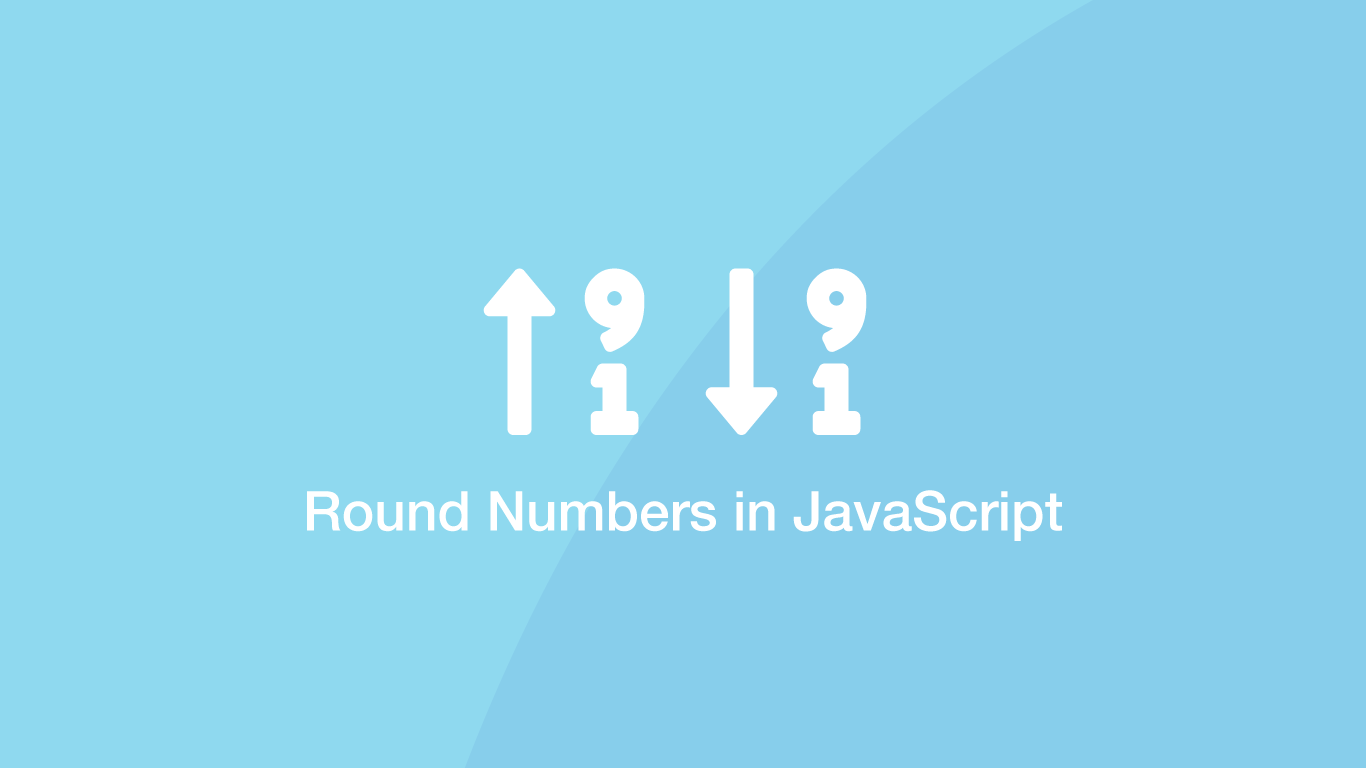# How to Round Decimals Up and Down in JavaScriptWhen working with numbers in JavaScript it is common to encounter decimals that need to be rounded to integers. Fortunately, JavaScript has native functions that can round numbers to the nearest integer, or always up or down to a whole number.

In this tutorial, we will learn how to round decimals up and down in JavaScript with examples.

## Round to the Nearest Integer

In most cases, we will want to simply round a number with decimal places to the nearest integer. This can be done with the `Math.round()` method. To use it pass the decimal inside the `()` (parenthesis).

``````var decimal = 1.645;

var integer = Math.round(decimal);

console.log(integer);
``````
``````2
``````

The nearest integer to `1.645` is `2` and when we put that decimal into `Match.round()` `2` was indeed returned.

## Round Decimals Up

If you need to always round a number up, regardless of whether it is the nearest integer or not use the `Match.ceil()` method.

``````var decimal = 1.4;

var integer = Math.ceil(decimal);

console.log(integer);
``````
``````2
``````

The nearest integer to `1.4` is not `2` but `ceil()` rounded up to `2` anyway.

## Round Decimals Down

To always round a decimal down to an integer regardless of whether it is the closest integer use the `Math.floor()` method.

``````var decimal = 1.842;

var integer = Math.floor(decimal);

console.log(integer);
``````
``````1
``````

In the example above `1.842` was rounded down to `1`.

## Round to a Fixed Number of Decimal Places

It is possible to round decimals to a fixed number of decimal places using the `toFixed()` method. Pass in the decimal place at which the number should be rounded to inside the `()` (parenthesis).

``````var decimal = 1.846;

var integer = decimal.toFixed(2);

console.log(integer);
``````
``````1.85
``````

In the above example, we are rounding the number `1.846` to `2` decimal places. Essentially it is rounding 46 to 50 and returning `1.85` as the result.

## Conclusion

You now know how to round numbers in JavaScript to whole numbers and to fixed decimal places.

#### Related Tutorials### How to Round Numbers Up and Down in Python

September 28, 2020### How to Convert a String to a Number in JavaScript

August 20, 2020### Truncate Float in Python

June 29, 2021### Round a Number to 2 Decimal Places in JavaScript

November 24, 2021### How to Always Round-Up a Number in Python

November 22, 2021### How to Generate Random Numbers in JavaScript

September 13, 2020